Documentation

# transreduction

Transitive reduction

## Syntax

``H = transreduction(G)``

## Description

example

````H = transreduction(G)` returns the transitive reduction of graph `G` as a new graph, `H`. The nodes in `H` are the same as those in `G`, but `H` has different edges. `H` contains the fewest number of edges such that if there is a path from node `i` to node `j` in `G`, then there is also a path from node `i` to node `j` in `H`.```

## Examples

collapse all

Create and plot a complete graph of order four.

```G = digraph([1 1 1 2 2 2 3 3 3 4 4 4],[2 3 4 1 3 4 1 2 4 1 2 3]); plot(G)```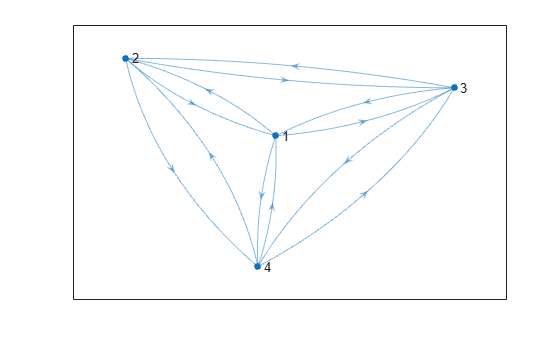Find the transitive reduction and plot the resulting graph. Since the reachability in a complete graph is extensive, there are theoretically several possible transitive reductions, as any cycle through the four nodes is a candidate.

```H = transreduction(G); plot(H)```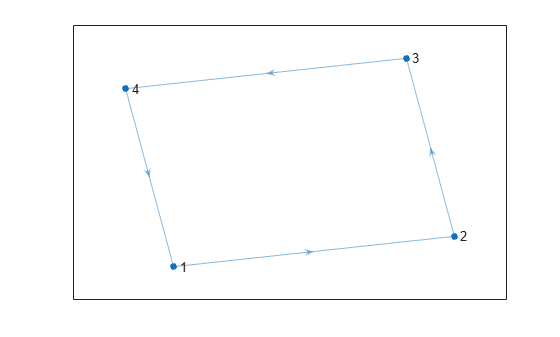Two graphs with the same reachability also have the same transitive reduction. Therefore, any cycle of four nodes produces the same transitive reduction as `H`.

Create a directed graph that contains a different four node cycle: (1,3,4,2,1).

```G1 = digraph([1 3 4 2],[3 4 2 1]); plot(G1)```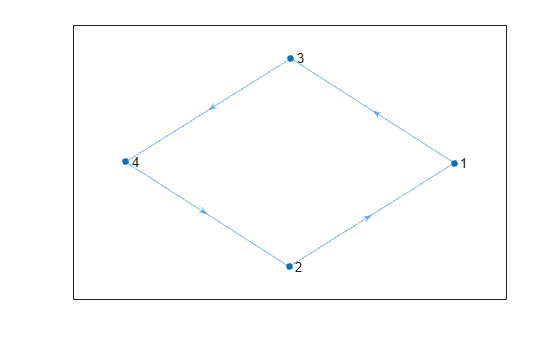Find the transitive reduction of `G1`. The cycle in `G1` is reordered so that the transitive reductions `H` and `H1` have the same cycle, (1,2,3,4,1).

```H1 = transreduction(G1); plot(H1)```Create and plot a directed acyclic graph.

```s = [1 1 1 1 2 3 3 4]; t = [2 3 4 5 4 4 5 5]; G = digraph(s,t); plot(G)```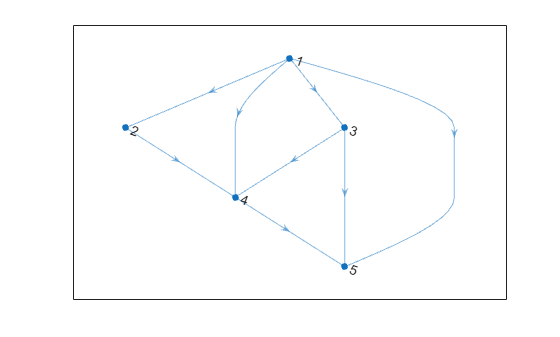Confirm that `G` does not contain any cycles.

`tf = isdag(G)`
```tf = logical 1 ```

Find the transitive reduction of the graph. Since the graph does not contain cycles, the transitive reduction is unique and is a subgraph of `G`.

```H = transreduction(G); plot(H)```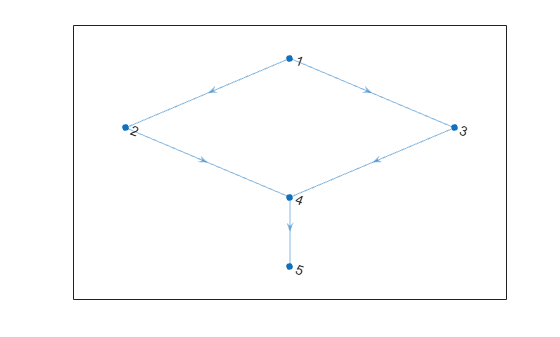## Input Arguments

collapse all

Input graph, specified as a `digraph` object. Use `digraph` to create a directed graph object.

Example: `G = digraph([1 2],[2 3])`

## Output Arguments

collapse all

Transitive reduction of `G`, returned as a `digraph` object. The table `G.Nodes` is copied to `H`, but any properties in `G.Edges` are dropped. `H` might contain new edges not present in `G`.

`H` contains the fewest number of edges that still preserve the reachability of graph `G`. In other words, `transclosure(H)` is the same as `transclosure(G)`.

If `isdag(G)` is `true`, then `H` is unique and is a subgraph of `G`.

collapse all

### Transitive Reduction

The transitive reduction of graph `G` is the graph with the fewest edges that still shares the same reachability as `G`. Therefore, of all the graphs that have the same transitive closure as `G`, the transitive reduction is the one with the fewest edges. If two directed graphs have the same transitive closure, they also have the same transitive reduction.